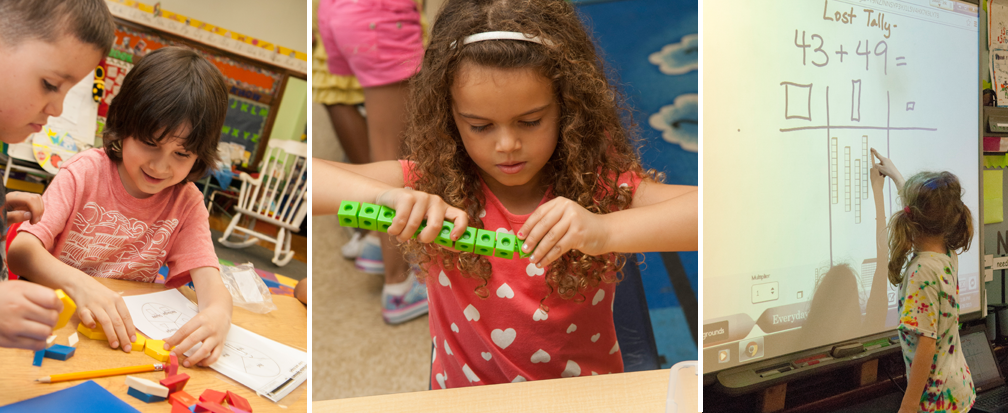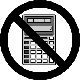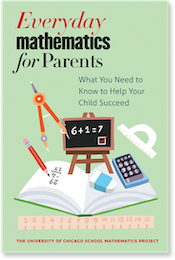## Calculator Use

### How and why does Everyday Mathematics encourage the use of calculators?

Based on research that has shown calculator use can enhance cognitive gains in the areas of number sense, conceptual development and visualization, the National Council of Teachers of Mathematics recommends the integration of calculators into mathematics programs for all grade levels. Everyday Mathematics offers appropriate applications for the calculator at each grade level.

In the Everyday Mathematics program, emphasis is placed on using the calculator as a tool for learning mathematics. In kindergarten, for example, calculators add a visual dimension to oral counting routines as children count forwards and backwards by 1s, 2s, 5s, and 10s. Seeing the numbers on the calculator display as they count also helps children learn written number sequences.

In first and later grades, students play a calculator game called Beat the Calculator. This game challenges students to develop an automatic recall of the basic facts, and demonstrates why it is better to develop quick mental math skills instead of always relying on a calculator. The program also includes a number of calculator games that are designed to provide practice with place value and problem-solving skills.

The student materials also include exercises for which it would be inappropriate to use calculators. These are clearly marked with "no calculator" signs:The University of Chicago School Mathematics Project

University of Chicago Press